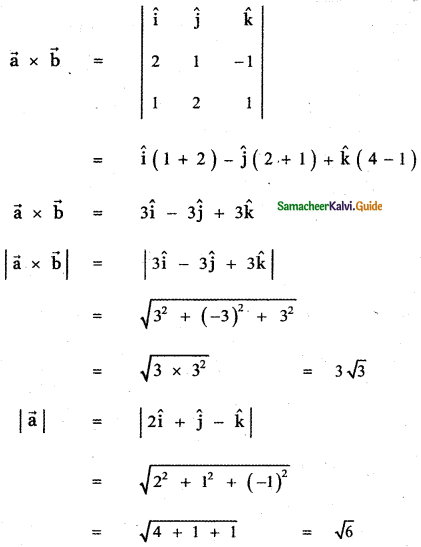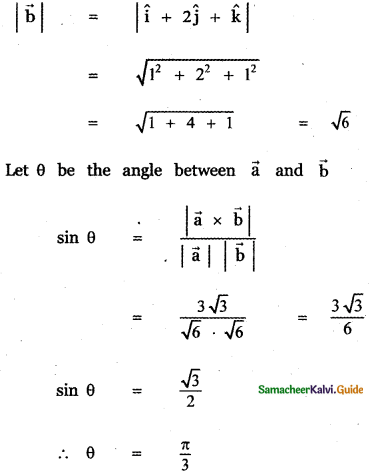Tamilnadu State Board New Syllabus Samacheer Kalvi 11th Maths Guide Pdf Chapter 8 Vector Algebra – I Ex 8.4 Text Book Back Questions and Answers, Notes.

## Tamilnadu Samacheer Kalvi 11th Maths Solutions Chapter 8 Vector Algebra – I Ex 8.4

Question 1.
Find the magnitude of $$\vec{a}$$ × $$\vec{b}$$ if $$\vec{a}$$ = 2î + ĵ + 3k̂ and $$\vec{b}$$ = 3î + 5ĵ – 2k̂
The given vectors are $$\vec{a}$$ = 2î + ĵ + 3k̂
$$\vec{b}$$ = 3î + 5ĵ – 2k̂Question 2.
Show thatQuestion 3.
Find the vectors of magnitude 10√3 that are perpendicular to the plane which contains
î + 2ĵ + k̂ and î + 3ĵ + 4k̂
Let the given vectors be $$\vec{a}$$ = î + 2ĵ + k̂
$$\vec{b}$$ = î + 3ĵ + 4k̂Question 4.
Find the unit vectors perpendicular to each of the vectors $$\vec{a}$$ + $$\vec{b}$$ and $$\vec{a}$$ – $$\vec{b}$$, where $$\vec{a}$$ = î + ĵ + k̂ and $$\vec{b}$$ = î + 2ĵ + 3k̂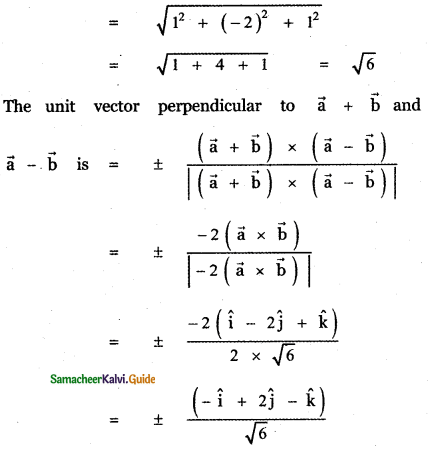Question 5.
Find the area of the parallelogram whose two adjacent sides are determined by the vectors î + 2ĵ + 3k̂ and 3î – 2ĵ + k̂Question 6.
Find the area of the triangle whose vertices are A(3, -1, 2), B(1, -1, -3), and C(4, -3, 1)
The given vertices of the triangle ABC are
A(3, -1, 2), B(1, -1, -3) and C(4, -3, 1)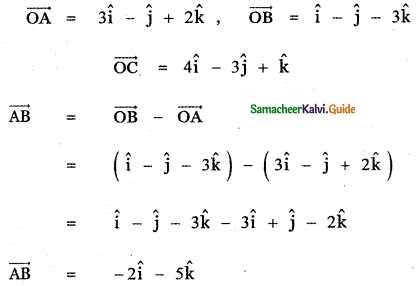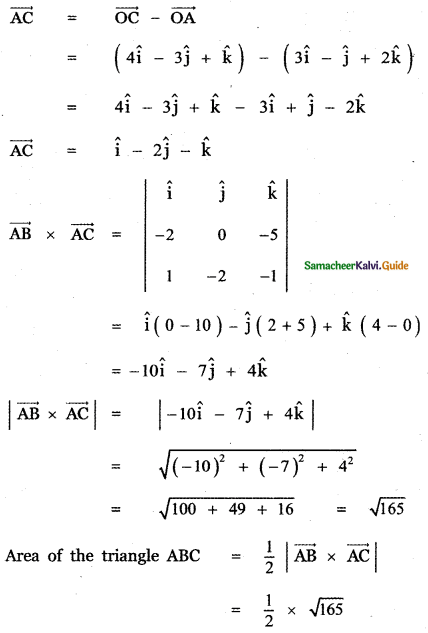Question 7.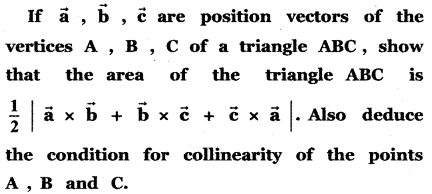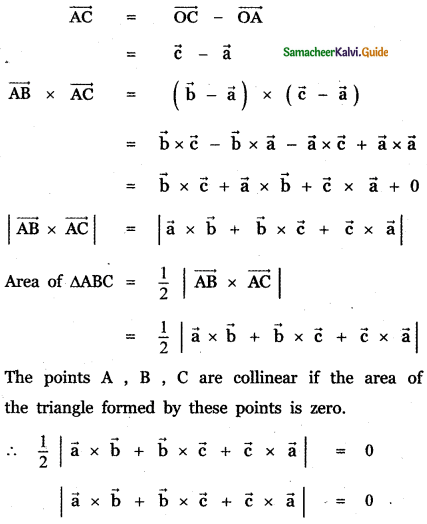Question 8.
For any vector $$\vec{a}$$ prove that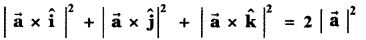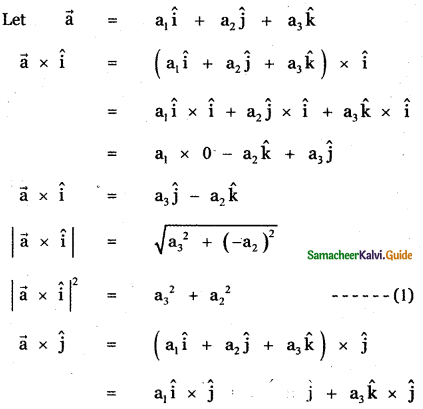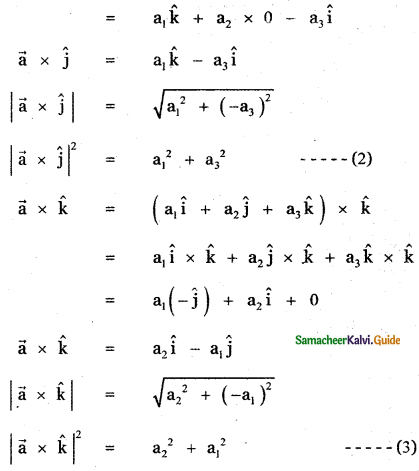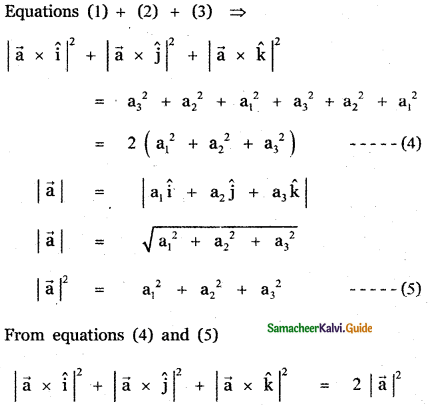Question 9.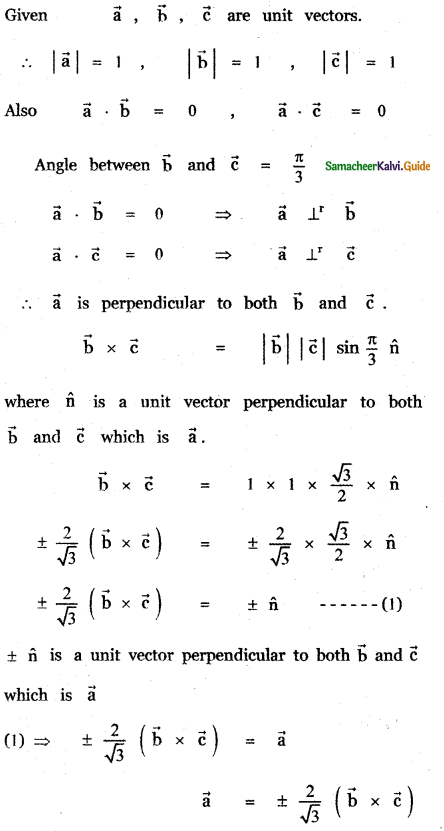Let the given vector be $$\vec{a}$$ = 2î + ĵ – k̂ and $$\vec{b}$$ = 2î + ĵ – k̂